# Monday, March 20th

Mauri Dovan First Day of Spring (2).mp3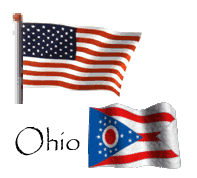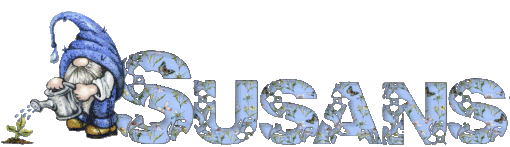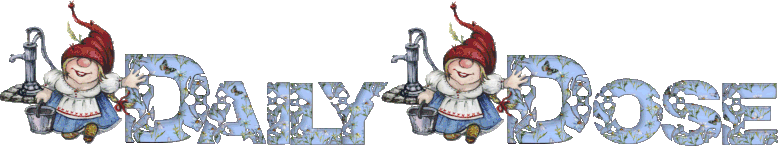Susan Kistler, Creator-Publisher
Since 2001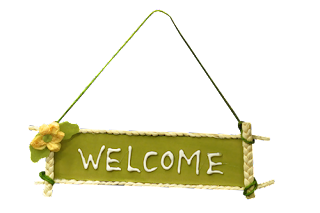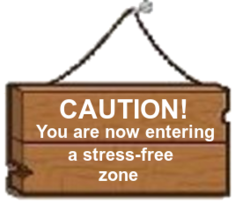Yes, things end,

but memories

last forever ....

Hold tight

to those ...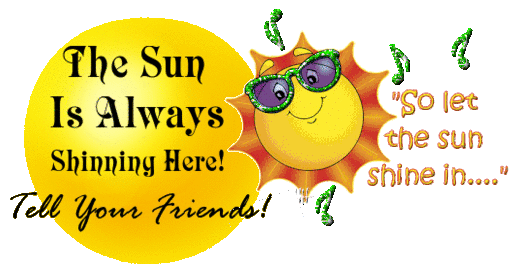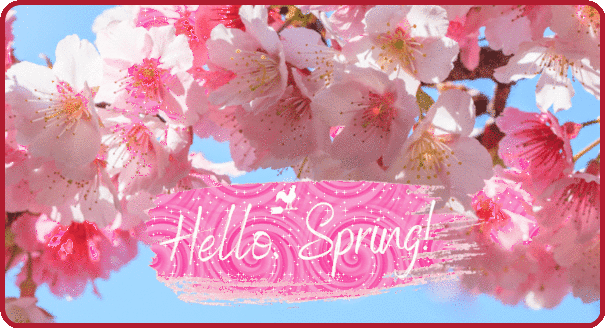2003 - The United States invade Iraq, assisted by
the United Kingdom, Australia and Poland.
1995 - Japanese terrorists release poisonous
gas in the Tokyo subway.
1969 - John Lennon and Yoko Ono marry.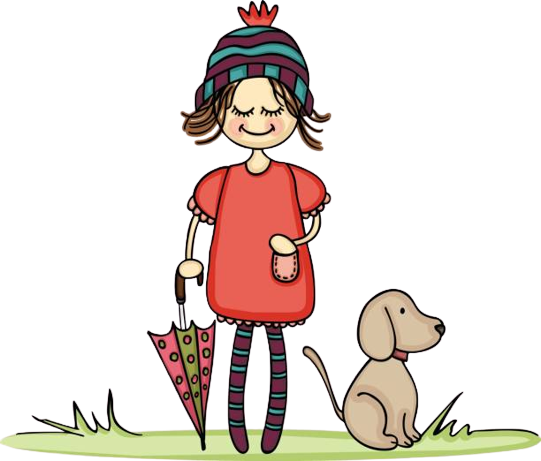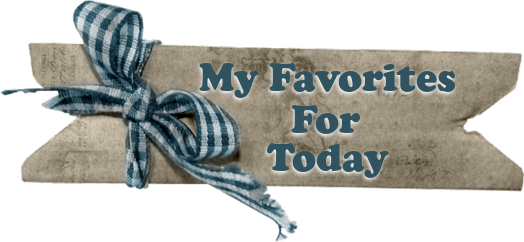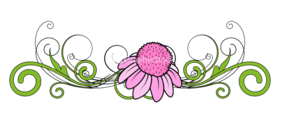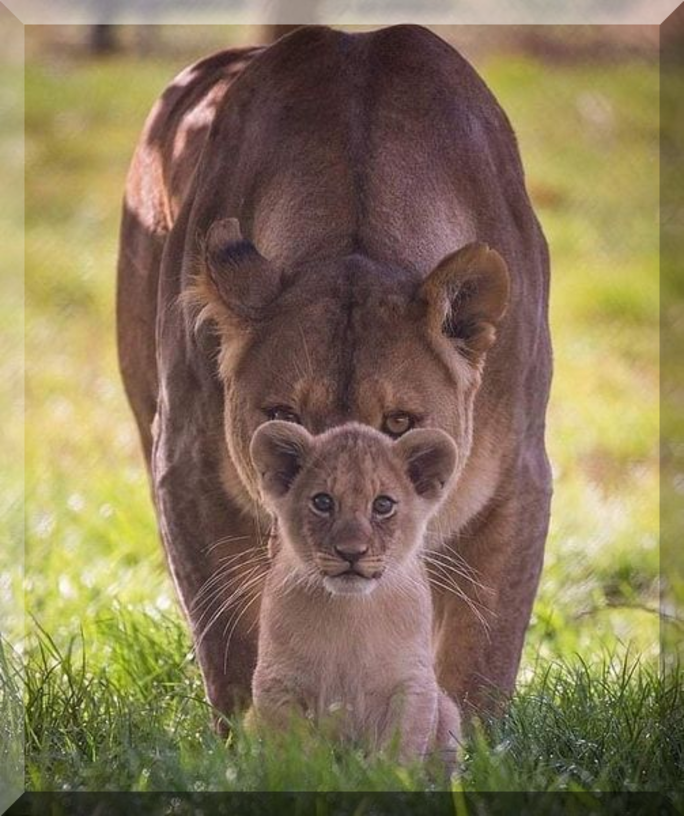Do not touch my baby!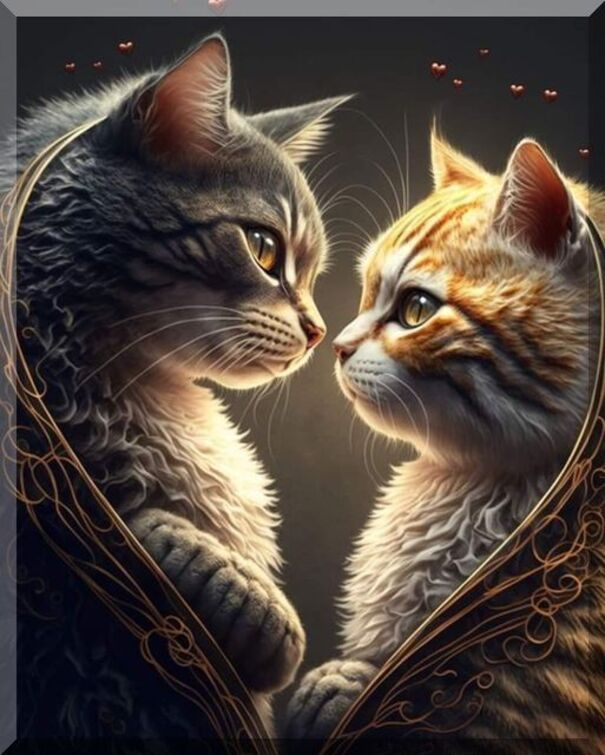^^^^^^^^^^^^^^^^^^^^^^^^^^^^^^^^^^^^^^^^^^^^^^^^^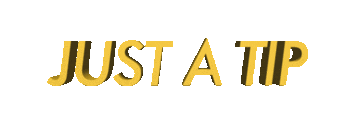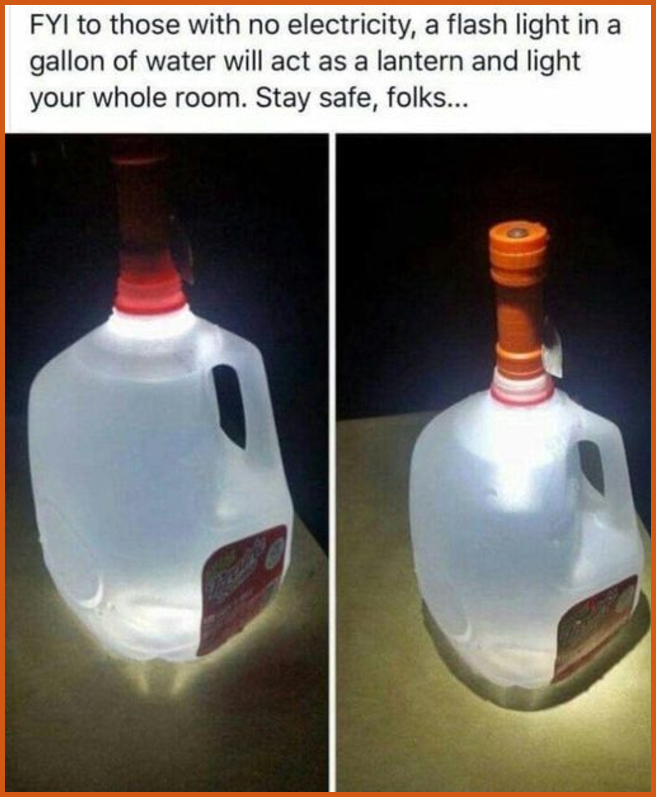I've never tried this, but the next gallon of
milk I empty - I think I'll try it and see if it works.

^^^^^^^^^^^^^^^^^^^^^^^^^^^^^^^^^^^^^^^^^^^^^^^^^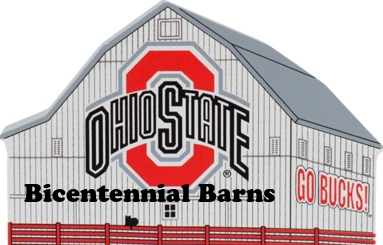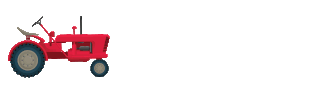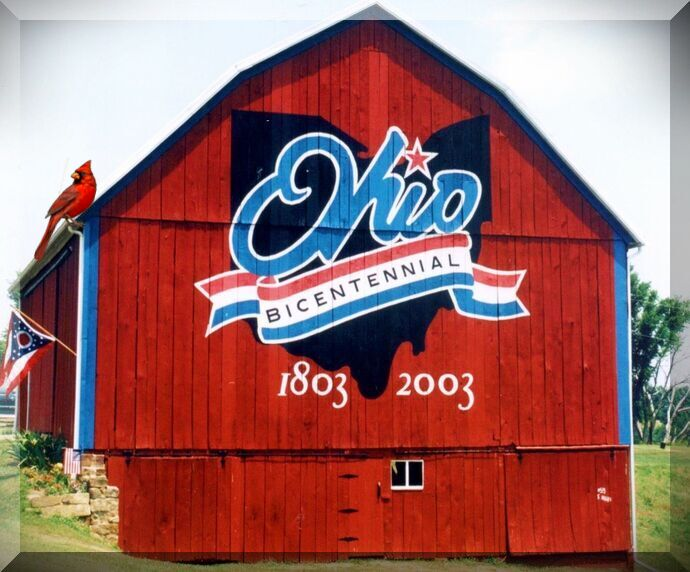^^^^^^^^^^^^^^^^^^^^^^^^^^^^^^^^^^^^^^^^^^^^^^^^^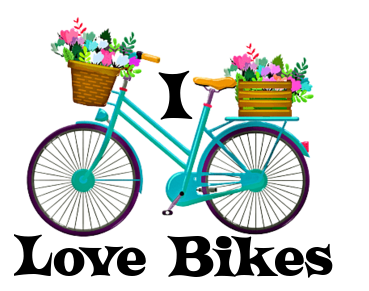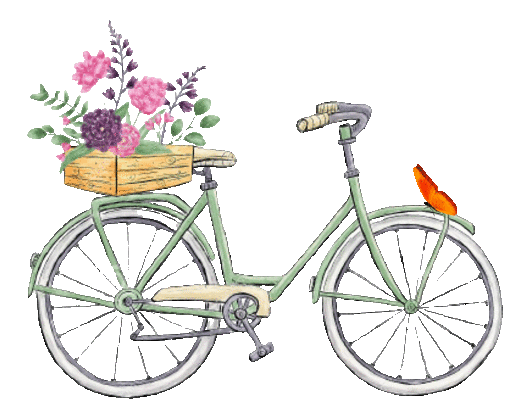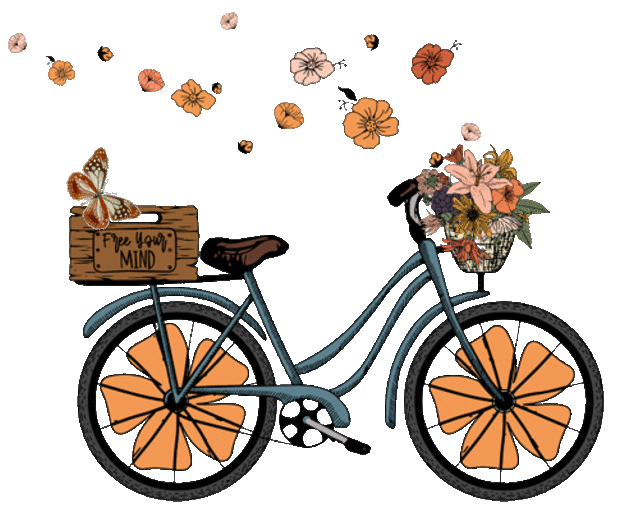^^^^^^^^^^^^^^^^^^^^^^^^^^^^^^^^^^^^^^^^^^^^^^^^^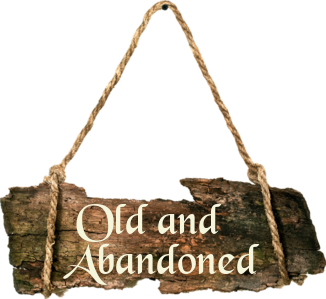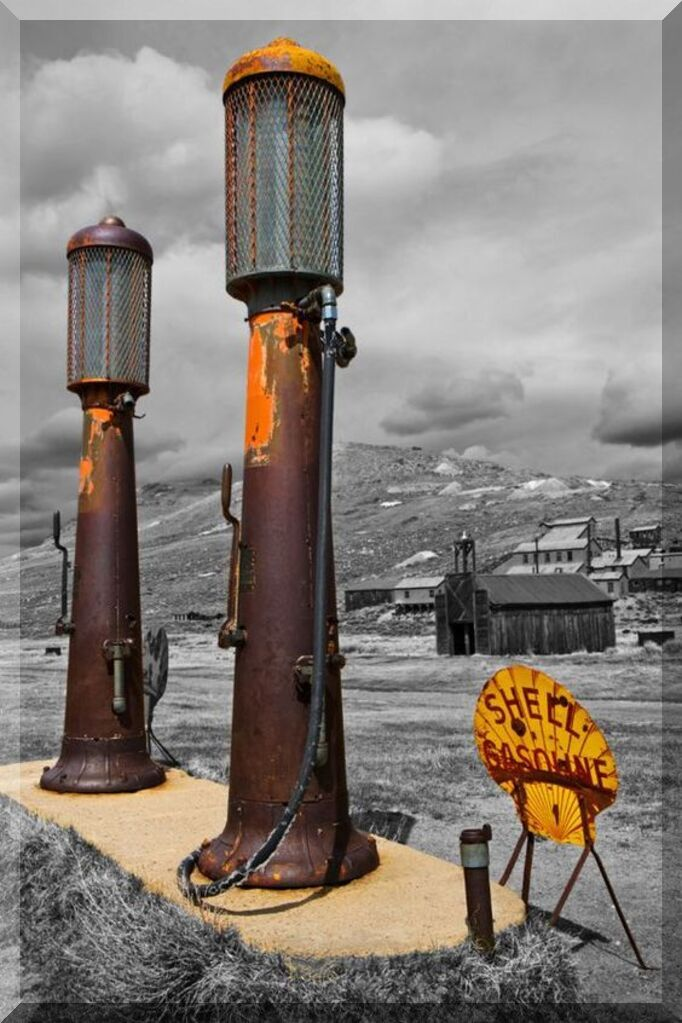^^^^^^^^^^^^^^^^^^^^^^^^^^^^^^^^^^^^^^^^^^^^^^^^^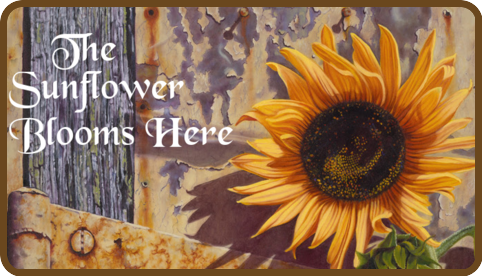Beginning today - Sunflowers!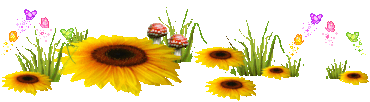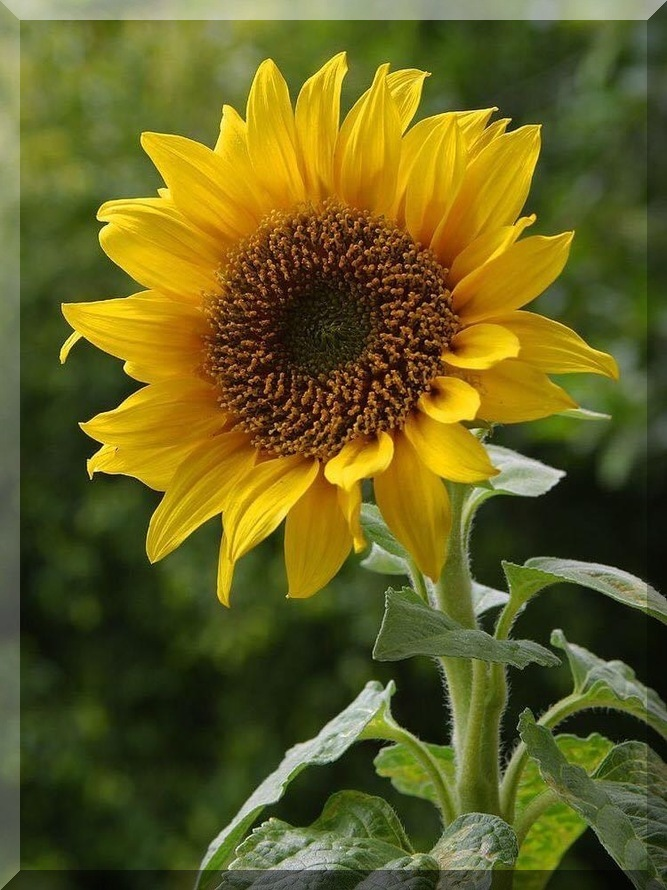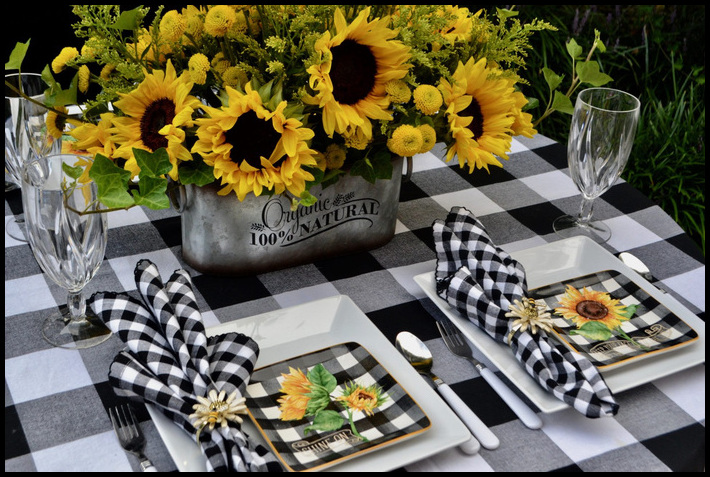^^^^^^^^^^^^^^^^^^^^^^^^^^^^^^^^^^^^^^^^^^^^^^^^^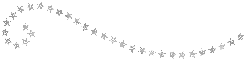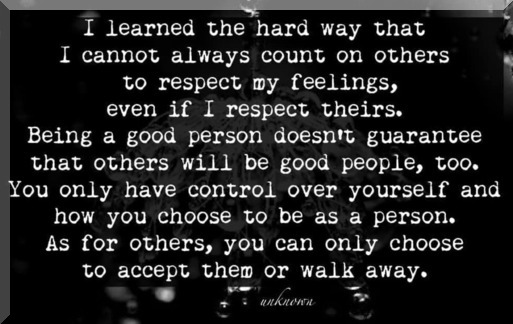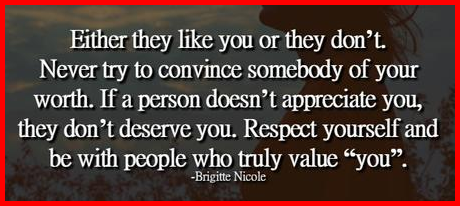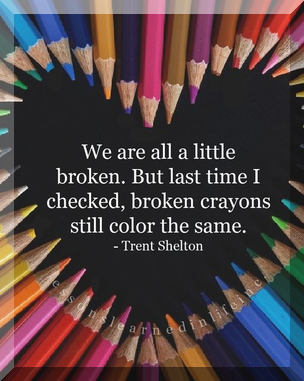^^^^^^^^^^^^^^^^^^^^^^^^^^^^^^^^^^^^^^^^^^^^^^^^^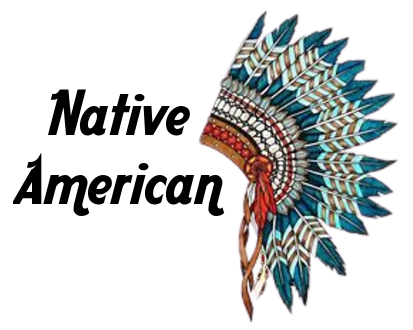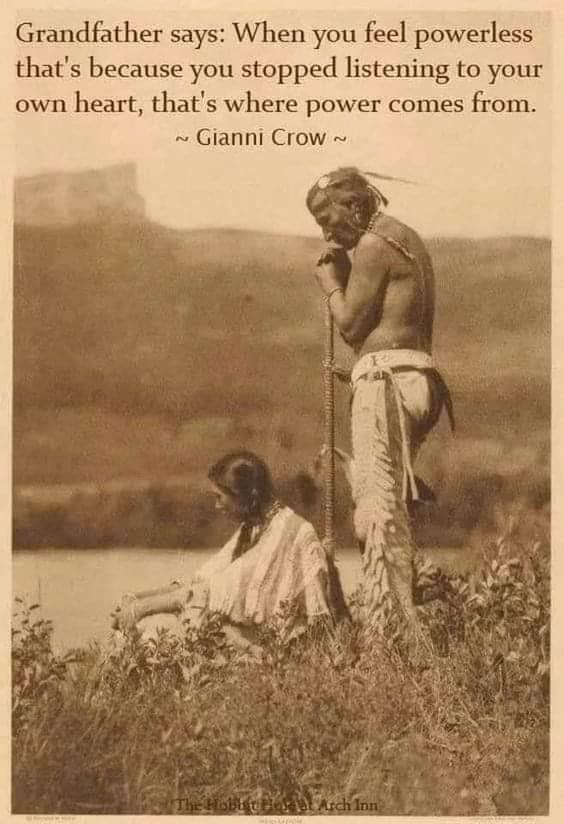^^^^^^^^^^^^^^^^^^^^^^^^^^^^^^^^^^^^^^^^^^^^^^^^^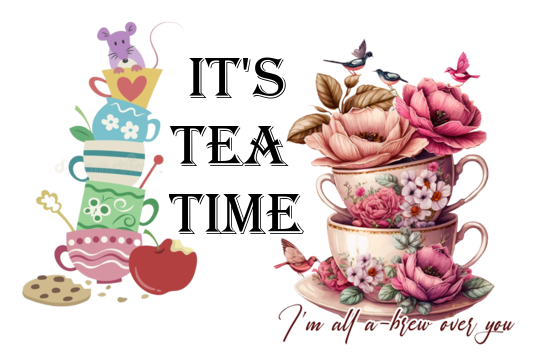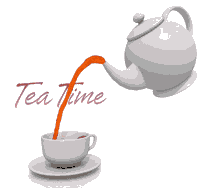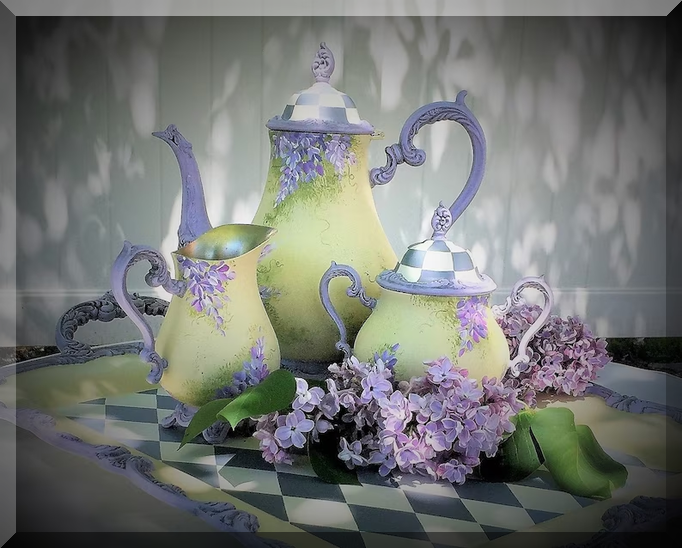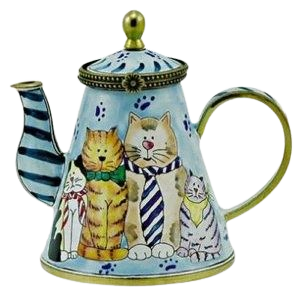^^^^^^^^^^^^^^^^^^^^^^^^^^^^^^^^^^^^^^^^^^^^^^^^^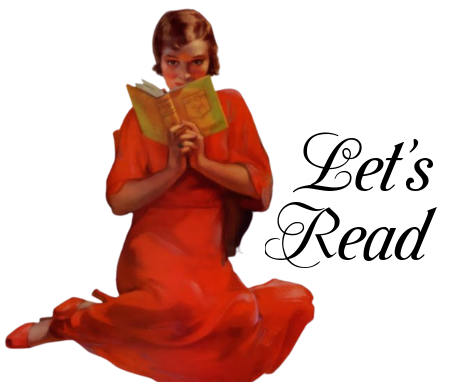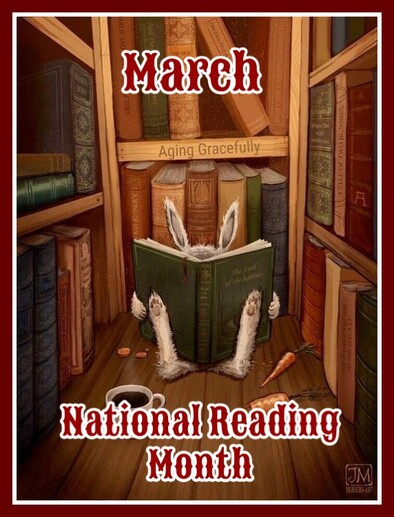In honor of Dr Suess' birthday, March is
designated as Nation Reading Month - a month
to motivate Americans of all ages to read every
day. Reading is fun and has many benefits,
regardless of your age. It's a key component
of education and professional development.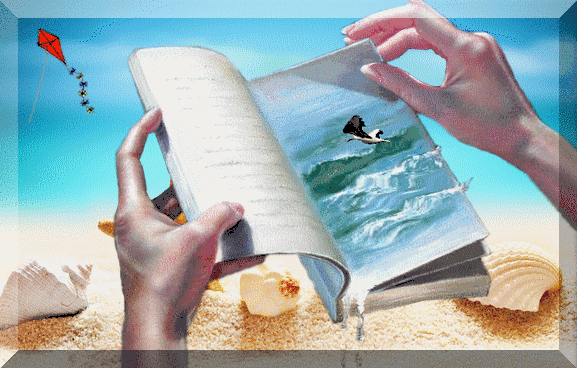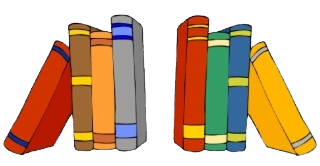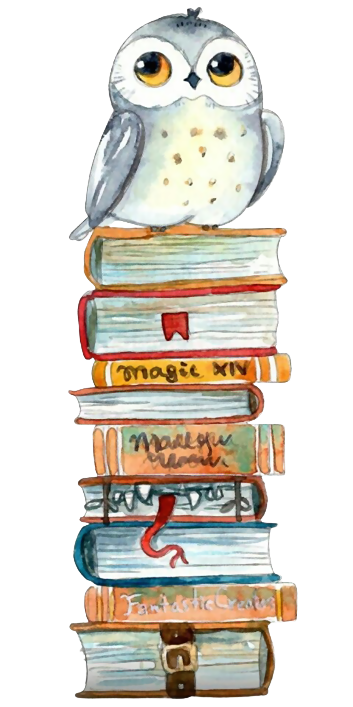^^^^^^^^^^^^^^^^^^^^^^^^^^^^^^^^^^^^^^^^^^^^^^^^^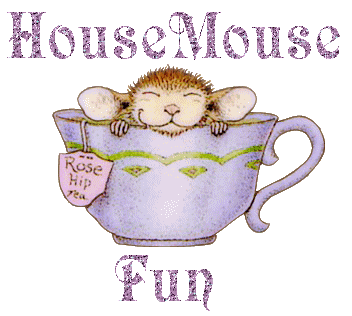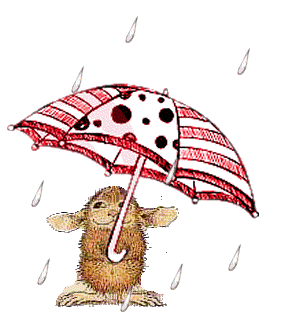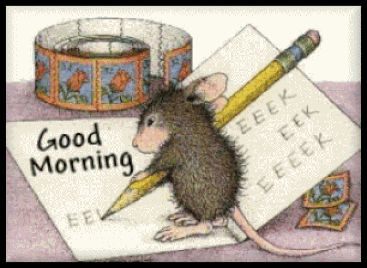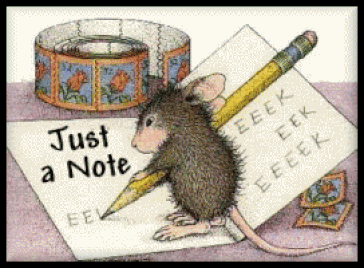^^^^^^^^^^^^^^^^^^^^^^^^^^^^^^^^^^^^^^^^^^^^^^^^^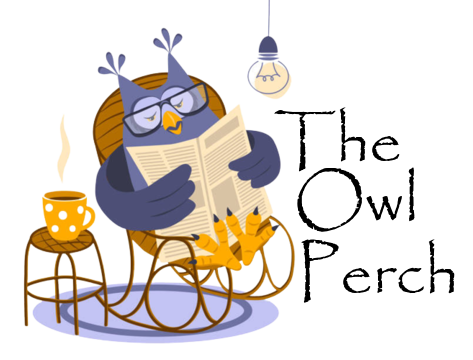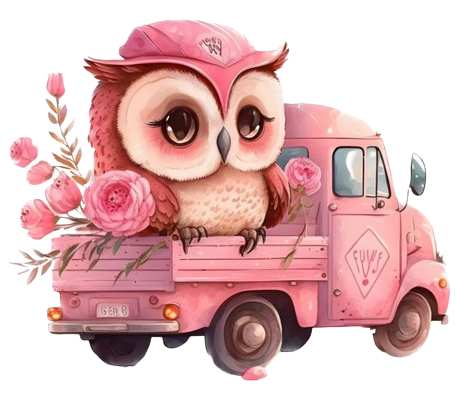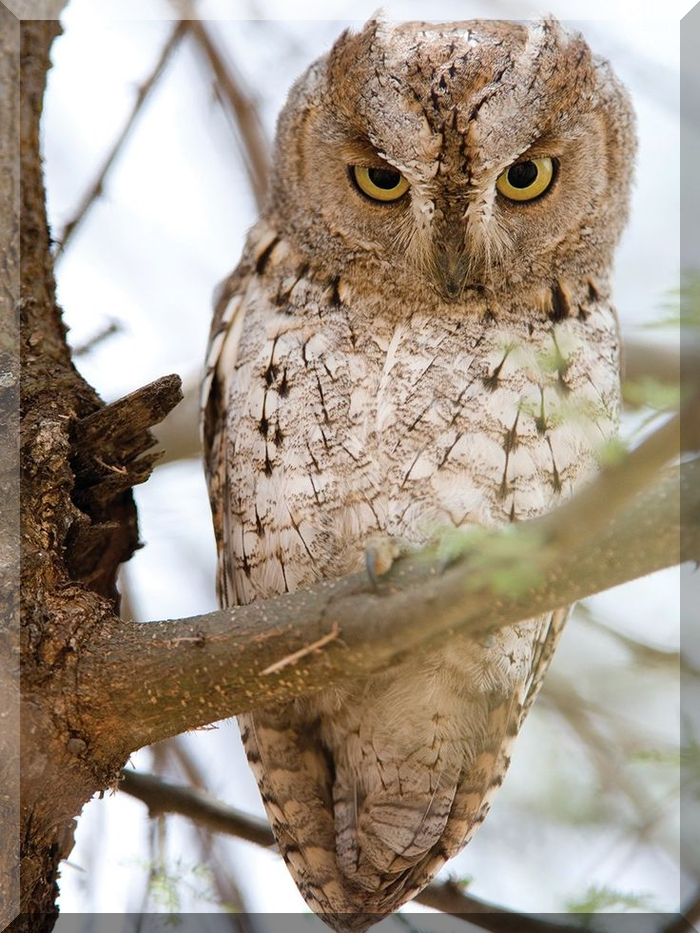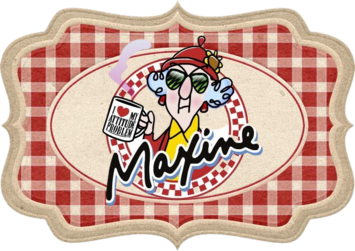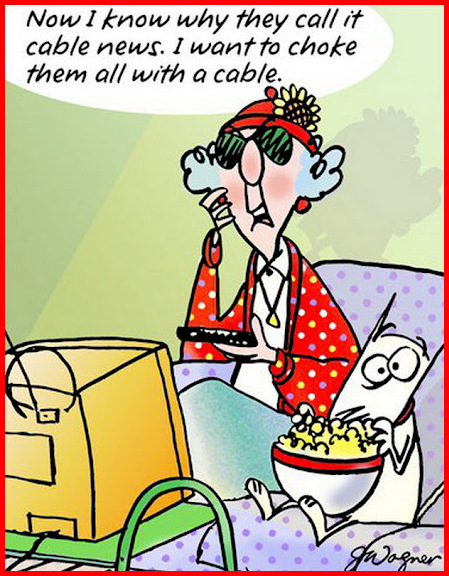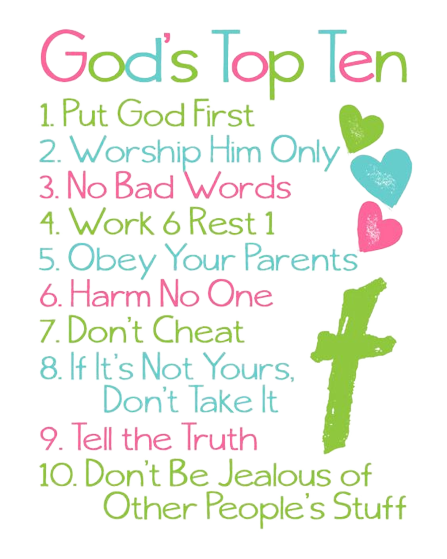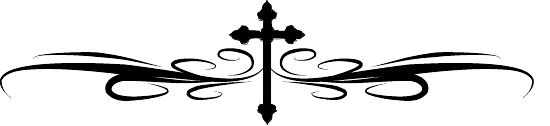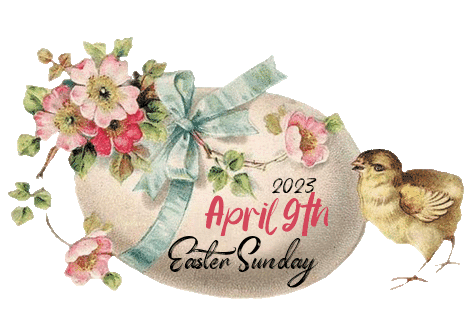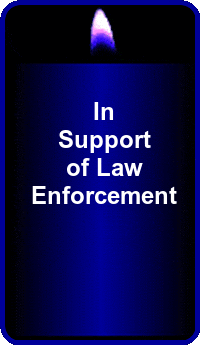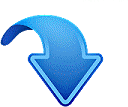I have taken time to include on my pages below.
I will appreciate if you do!
Thank you.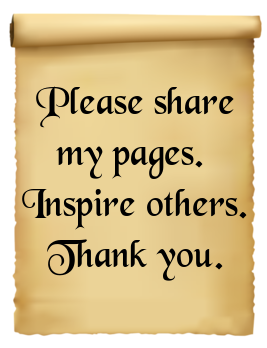Sharing a wonderful blog/website
https://tattysthingies.blogspot.com/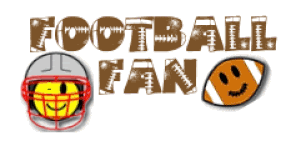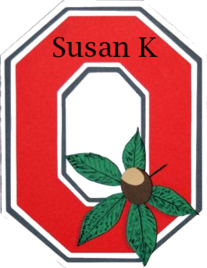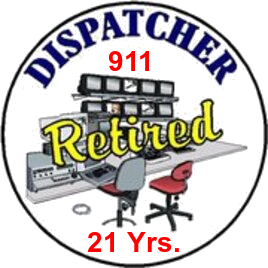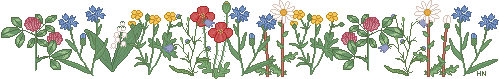Hello, and I would like to let you know that I do accept
donations to help pay for this website. Donations do help
me a lot and are very appreciated. The donate button above
will lead you to PayPal, a safe and easy way to donate.
Not comfortable with PayPal, simply email me and I can
send you my address for mailing. Thank you.The below site will help animals and others. Please
do visit and see how you can help by "just clicking"!
It only takes a minute. I used to have this on my
pages and quit for one reason or another and I
very much wanted to do it again. We all CAN make
a difference. VISIT TODAY!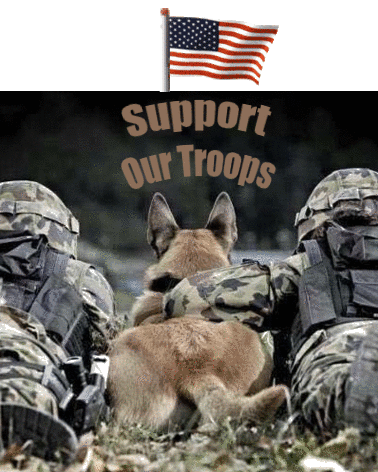The below map shows
location of visitors